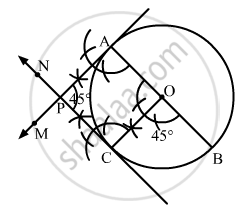Share

# Draw a Pair of Tangents to a Circle of Radius 4.5 Cm, Which Are Inclined to Each Other at an Angle of 45°. - Mathematics

Course

#### Question

Draw a pair of tangents to a circle of radius 4.5 cm, which are inclined to each other at an angle of 45°.

#### Solution

Steps of Construction
Step 1. Draw a circle with centre O and radius 4.5 cm.
Step 2. Draw any diameter AOB of the circle.
Step 3. Construct ∠BOC = 45º such that radius OC cuts the circle at C.
Step 4. Draw AM ⊥ AB and CN ⊥ OC. Suppose AM and CN intersect each other at P.Here, AP and CP are the pair of tangents to the circle inclined to each other at an angle of 45º.

Is there an error in this question or solution?